# CS 109A/AC 209A/STAT 121A Data Science: Homework 2¶

Harvard University
Fall 2016
Instructors: W. Pan, P. Protopapas, K. Rader
Due Date: Wednesday, September 21st, 2016 at 11:59pm

Download the IPython notebook as well as the data file from Vocareum and complete locally.

To submit your assignment, in Vocareum, upload (using the 'Upload' button on your Jupyter Dashboard) your solution to Vocareum as a single notebook with following file name format:

last_first_CourseNumber_HW2.ipynb

where CourseNumber is the course in which you're enrolled (CS 109a, Stats 121a, AC 209a). Submit your assignment in Vocareum using the 'Submit' button.

Avoid editing your file in Vocareum after uploading. If you need to make a change in a solution. Delete your old solution file from Vocareum and upload a new solution. Click submit only ONCE after verifying that you have uploaded the correct file. The assignment will CLOSE after you click the submit button.

Problems on homework assignments are equally weighted. The Challenge Question is required for AC 209A students and optional for all others. Student who complete the Challenge Problem as optional extra credit will receive +0.5% towards your final grade for each correct solution.

Import libraries

In :
import numpy as np
import pandas as pd
import random
import sklearn as sk
from sklearn.neighbors import KNeighborsRegressor as KNN
from sklearn.cross_validation import train_test_split as sk_split
from sklearn.linear_model import LinearRegression as Lin_Reg
import time
import matplotlib
import matplotlib.pyplot as plt
%matplotlib inline
import math
import operator


## Problem 0: Basic Information¶

[Hagmann, Tim]

[CS 109a]

### Part (c): Who did you work with?¶

[First and Land names of students with whom you have collaborated]

## Problem 1: Inside the Models in Scikit-learn¶

In this problem, we will be implementing K-Nearest Neighbour and simple linear regression for predicting a quantitative variable. We will compare the performance of our implementation with those of Scikit-learn (sklearn).

The datasets required for this problem is in the dataset directory. Each file in the dataset directory contains a one-dimensional data set, with the first column containing the independent variable X, and the second column containing the dependent variable Y.

### Part (a): Implement the models by hand¶

In this part you may not use sklearn for any task.

In the following, you may use numpy arrays instead of pandas dataframes.

• Implement a funtion split, which satifies:
• input: an nx2 dataframe data, a float m
• return: an nx2 dataframe train and an nx2 dataframe test, consisting of m percent and 100 - m percent of the data, respectively.
• Implement K-Nearest Neighbour for predicting a quantitative variable. That is, write a function, knn_predict, that satisfies:
• input: an integer k, an n x 2 dataframe training set train, an n x 1 dataframe testing set test
• return: an nx2 dataframe, whose first column is that of test and whose second column is the predicted values.
• Implement linear regression for predicting a quantitative variable. That is, write a function linear_reg_fit that satisfies:
• input: an nx2 dataframe training set train
• return: the coefficients of the linear regression model - a float slope and a float intercept.
• Write a function linear_reg_predict that satisfies:
• input: an nx1 dataframe testing set test, as well as the coefficients of the linear regression model
• return: an nx2 dataframe, whose first column is that of test and whose second column is the predicted values.
• Implement a function score that satisfies:
• input: an nx2 dataframe predicted, an nx2 dataframe actual
• return: R^2 coefficient of the fit of the predicted values.

Solution:

In :
## Functions
#-----------
def split(data, m):
# Find the number of records dataframe
length = data.shape

# Build a list of integer indices for records in dataframe
indices = range(length)

# Randomly shuffle the indices
np.random.shuffle(indices)

# Take 70% of the shuffled list of indices for training
train_indices = indices[0:int(length * m/100)]

# Take the remainder the shuffled list of indices for testing
test_indices = indices[int(length * m/100):]

# Get the records for the training indices
train = data.iloc[train_indices, :]
# train = train.reset_index(drop=True)
# train['index'] = train_indices

# Get the records for the testing indices
test = data.iloc[test_indices, :]
# test = test.reset_index(drop=True)
# test['index'] = test_indices

return train, test

## KNN
#-----
def euclideanDistance(instance1, instance2, length):
distance = 0
for x in range(length):
distance += pow((instance1[x] - instance2[x]), 2)
return math.sqrt(distance)

def getNeighbors(trainingSet, testInstance, k):
distances = []
length = len(testInstance)-1
for x in range(len(trainingSet)):
dist = euclideanDistance(testInstance, trainingSet[x], length)
distances.append((trainingSet[x], dist))
distances.sort(key=operator.itemgetter(1))
neighbors = []
for x in range(k):
neighbors.append(distances[x])
return neighbors

def getResponse(neighbors):
n = len(neighbors)
num_vec = np.zeros(n)
for i in range(n):
num_vec[i] = neighbors[i]
return np.mean(num_vec)

def knn_predict(train, test, k):
# prepare data
trainingSet=[]
testSet=[]
predictions=[]
actual=[]

for i in range(len(train)):
trainingSet.append([train["x"].values[i], train["y"].values[i]])
for i in range(len(test)):
testSet.append([test["x"].values[i], test["y"].values[i]])

for x in range(len(testSet)):
neighbors = getNeighbors(trainingSet, testSet[x], k)
result = getResponse(neighbors)
y = testSet[x]
predictions.append(result)
actual.append(y)

d = {'actual': pd.Series(actual),
'prediction': pd.Series(predictions)}
return pd.DataFrame(d)

## Linear regression
#-------------------
def linear_reg_fit(train):
# get x and y vars
X = train['x'].values
Y = train['y'].values

# Estimate the coefficients
beta1 = np.sum((X-np.mean(X)) * (Y-np.mean(Y)))/np.sum( (X-np.mean(X))**2)
beta0 = np.mean(Y) - beta1*np.mean(X)
return beta0, beta1

def linear_reg_predict(test, beta0, beta1):
X = test['x'].values
Y = test['y'].values
Y_Hat = beta0 + beta1 * X
d = {'actual': pd.Series(Y),
'prediction': pd.Series(Y_Hat)}
return pd.DataFrame(d)

## Scoring
#---------
actual = test['actual'].values
prediction = test['prediction'].values
return np.sum((actual-prediction)**2)

# Calculate TSS
def TSS(test):
actual = test['actual'].values
return np.sum((actual - np.mean(actual))**2)

# Calculate R^2
def score(test):
return (1 - (RSS(test) / TSS(test)))


### Part (b): Compare with sklearn¶

• Load the contents of dataset_1_full.txt into a pandas dataframe, or numpy array.
• Use your functions from Part (a) to split the data into training and testing sets (70-30). Evaluate how KNN and linear regression each perform on this dataset.
• Use sklearn to split the data into training and testing sets (70-30). Use sklearn to evaluate how KNN and linear regression each perform on this dataset.
• Use Python's time library to measure how well your implementations compare with that of sklearn. What can you do (algorithmically or codewise) to make your implementation faster or more efficient?

Solution:

In :
# Read data into pandas df

# Size of data frame
print 'number of total observations:', df.shape, "\n"

# Split data
np.random.seed(111) # Set seed
train, test = split(data=df, m=70)

# Print first 5 rows of dataframe
print 'number of training observations:', train.shape, "\n"
print 'number of test observations:', test.shape

number of total observations: 500

x         y
292  0.102934  2.091309
337  0.206823  0.816355
430  0.979152  5.209166
399  0.661935  3.989140
380  0.042114 -0.006533
number of training observations: 350

x         y
245  0.449535  2.477697
392  0.843549  3.624430
232  0.682815  1.116077
315  0.547662  3.139188
424  0.357974  1.787528
number of test observations: 150

In :
# Linear regression
start = time.time()
beta0, beta1 = linear_reg_fit(train)
df_myfun_linreg = linear_reg_predict(test, beta0, beta1)
end = time.time()

print "R^2 of my linear regression algorithm:", round(score(df_myfun_linreg), 4)
print "Time elapsed: ", "%s seconds " % (end - start), "\n"

# KNN
start = time.time()
df_myfun_knn = knn_predict(train, test, 10)
end = time.time()

print "R^2 of my knn algorithm:", round(score(df_myfun_knn), 4)
print "Time elapsed: ", "%s seconds " % (end - start), "\n"

R^2 of my linear regression algorithm: 0.3846
Time elapsed:  0.000999927520752 seconds

R^2 of my knn algorithm: 0.3351
Time elapsed:  0.108999967575 seconds


In :
## Sklearn KNN
#-------------
train, test = sk_split(df, test_size=0.3, random_state=111)

# get individual values
x_train = train.loc[:,'x'].values
x_train = x_train.reshape((len(x_train), 1))
y_train = train.loc[:,'y'].values
x_test = test.loc[:,'x'].values
x_test = x_test.reshape((len(x_test), 1))
y_test = test.loc[:,'y'].values

# fit knn model
start = time.time()
neighbours = KNN(n_neighbors=10)
neighbours.fit(x_train, y_train)

# predict y-values
predicted_y = neighbours.predict(x_test)

# score predictions
r = neighbours.score(x_test, y_test)
end = time.time()

print "R^2 of the sklearn knn algorithm:", round(r, 4)
print "Time elapsed: ", "%s seconds " % (end - start), "\n"

R^2 of the sklearn knn algorithm: 0.3419
Time elapsed:  0.00399994850159 seconds


In :
## Sklearn Linear regression
#----------------------------

# get individual values
x_train = train.loc[:,'x'].values
x_train = x_train.reshape((len(x_train), 1))
y_train = train.loc[:,'y'].values
x_test = test.loc[:,'x'].values
x_test = x_test.reshape((len(x_test), 1))
y_test = test.loc[:,'y'].values

# fit linear model
start = time.time()
regression = Lin_Reg()
regression.fit(x_train, y_train)

# predict y-values
predicted_y = regression.predict(x_test)

# score predictions
r = regression.score(x_test, y_test)
end = time.time()

print "R^2 of the sklearn linear regression algorithm:", round(r, 4)
print "Time elapsed: ", "%s seconds " % (end - start), "\n"

R^2 of the sklearn linear regression algorithm: 0.4018
Time elapsed:  0.00799989700317 seconds



** Comment: **
As can be seen above, the R^2 results are similar over the different algorithms. It is important to note, that there is a random component involved, i.e., among different runs the algorithms performe differently. However, what can be seen is that my implementation of the KNN algorithm is slower. In order to improve on that result vectorisation of parts of the algorithm could help, i.e., reduce the amount of loops. Furthermore, every time indexing, sorting or copying is done, valuable computation time is lost. It might also helpt to work on those points.

## Problem 2: Handling Missing Data¶

In this problem, we will be handling the problem of datasets with missing values. Clearly, we cannot simply remove entire rows or columns that contain missing values. In this problem, we explore two different ways to fill in missing values.

The datasets required for this problem is in the dataset directory. Each file in the dataset directory contains a one-dimensional data set, with the first column containing the independent variable X, and the second column containing the dependent variable Y.

The files dataset_1_missing.txt to dataset_6_missing.txt contains rows that are missing their y-values, where as dataset_1_full.txt to dataset_6_full.txt contain datasets with all y-values correctly filled in.

In this problem, you may not use sklearn or build-in pandas functions to directly fill in missing values. Usage of these libraries/pakcages for related tasks is fine.

### Part (a): Model Based Data Imputation¶

• Describe in detail how predictive models for data (like KNN and simple linear regression) can be used to fill in missing values in a data set.
• Implement your scheme. That is, write code (preferably a function fill or two functions fill_knn, fill_lin_reg), which takes an n x 2 dataframe or array with values missing in the 2nd column and fills in these values using KNN and linear regression.
• You need to, also, write code to evaluate the quality of the values you've filled in.

Solution:

In :
#--------  fill_knn
#input: missing_df (dataframe), full_df (dataframe), no_y_ind (indices of missing values),
#       with_y_ind (indices of non-missing values), k (integer)
#output: predicted_df (dataframe), r (float)

def fill_knn(missing_df, full_df, no_y_ind, with_y_ind, k):
#preparing data in array form
x_train = missing_df.loc[with_y_ind, 'x'].values
x_train = x_train.reshape((len(with_y_ind), 1))
y_train = missing_df.loc[with_y_ind, 'y'].values
x_test = missing_df.loc[no_y_ind, 'x'].values.reshape((len(no_y_ind), 1))
y_test = full_df.loc[no_y_ind, 'y'].values

#fit knn model
neighbours = KNN(n_neighbors=k)
neighbours.fit(x_train, y_train)

#predict y-values
predicted_y = neighbours.predict(x_test)

#score predictions
r = neighbours.score(x_test, y_test)

#fill in missing y-values
predicted_df = missing_df.copy()
predicted_df.loc[no_y_ind, 'y'] = pd.Series(predicted_y, index=no_y_ind)

return predicted_df, r

#--------  fill_ling_reg
#input: missing_df (dataframe), full_df (dataframe), no_y_ind (indices of missing values),
#       with_y_ind (indices of non-missing values), k (integer)
#output: predicted_df (dataframe), r (float)

def fill_lin_reg(missing_df, full_df, no_y_ind, with_y_ind):
#preparing data in array form
x_train = missing_df.loc[with_y_ind, 'x'].values.reshape((len(with_y_ind), 1))
y_train = missing_df.loc[with_y_ind, 'y'].values
x_test = missing_df.loc[no_y_ind, 'x'].values.reshape((len(no_y_ind), 1))
y_test = full_df.loc[no_y_ind, 'y'].values

#fit linear model
regression = Lin_Reg()
regression.fit(x_train, y_train)

#predict y-values
predicted_y = regression.predict(x_test)

#score predictions
r = regression.score(x_test, y_test)

#fill in missing y-values
predicted_df = missing_df.copy()
predicted_df.loc[no_y_ind, 'y'] = pd.Series(predicted_y, index=no_y_ind)

return predicted_df, r



### Part (b): Which Model is Better?¶

• For datasets dataset_1_missing.txt to dataset_6_missing.txt, compare the result of filling in the missing values using KNN and linear regression, using both the R^2 coefficient as well as data visualization (the correct y-values are contained in dataset_1_full.txt to dataset_6_full.txt)..
• Use your analysis to form conjectures regarding the conditions under which KNN performs better than linear regression, under which linear regression performs better than KNN and under which both perform equally (well or poorly). Explain in detail exactly what might cause each model to fail or perform well.
• Using dataset_1_missing.txt, explain the impact of the choice of $k$ on the performance of KNN.

Use numerical analysis and data visualization to support every part of your argument.

Solution:

In :
#--------  plot_missing
#input: ax1 (axes), ax2 (axes),
#       predicted_knn (nx2 dataframe with predicted vals), r_knn (float),
#       predicted_lin (nx2 dataframe with predicted vals), r_lin (float),
#       k (integer),
#       no_y_ind (indices of rows with missing y-values),
#       with_y_ind (indices of rows with no missing y-values)
#output: ax1 (axes), ax2 (axes)

def plot_missing(ax1, ax2, predicted_knn, r_knn, predicted_lin, r_lin, k, no_y_ind, with_y_ind):
ax1.scatter(predicted_knn.loc[with_y_ind]['x'].values,
predicted_knn.loc[with_y_ind]['y'].values,
color='darkblue', alpha = 0.5)

ax1.scatter(predicted_knn.loc[no_y_ind]['x'].values,
predicted_knn.loc[no_y_ind]['y'].values,
color='darkred', alpha = 0.5)

ax1.set_title('KNN, R^2:' + str(r_knn))

ax2.scatter(predicted_lin.loc[with_y_ind]['x'].values,
predicted_lin.loc[with_y_ind]['y'].values,
color='darkblue', alpha = 0.5)

ax2.scatter(predicted_lin.loc[no_y_ind]['x'].values,
predicted_lin.loc[no_y_ind]['y'].values,
color='darkgreen', alpha = 0.5)

ax2.set_title('Lin Reg, R^2:' + str(r_lin))

return ax1, ax2

In :
#number of neighbours
k=10

#plot predicted points
fig, ((ax1, ax2), (ax3, ax4), (ax5, ax6)) = plt.subplots(3, 2, figsize=(15, 10))

no_y_ind = missing_df[missing_df['y'].isnull()].index
with_y_ind = missing_df[missing_df['y'].notnull()].index

predicted_knn, r_knn = fill_knn(missing_df,
full_df,
no_y_ind,
with_y_ind,
k)

predicted_lin, r_lin = fill_lin_reg(missing_df,
full_df,
no_y_ind,
with_y_ind)

ax5, ax6 = plot_missing(ax5,
ax6,
predicted_knn, r_knn,
predicted_lin, r_lin,
k,
no_y_ind,
with_y_ind)

no_y_ind = missing_df[missing_df['y'].isnull()].index
with_y_ind = missing_df[missing_df['y'].notnull()].index

predicted_knn, r_knn = fill_knn(missing_df,
full_df,
no_y_ind,
with_y_ind,
k)

predicted_lin, r_lin = fill_lin_reg(missing_df,
full_df,
no_y_ind,
with_y_ind)

ax1, ax2 = plot_missing(ax1,
ax2,
predicted_knn, r_knn,
predicted_lin, r_lin,
k,
no_y_ind,
with_y_ind)

no_y_ind = missing_df[missing_df['y'].isnull()].index
with_y_ind = missing_df[missing_df['y'].notnull()].index

predicted_knn, r_knn = fill_knn(missing_df,
full_df,
no_y_ind,
with_y_ind,
k)

predicted_lin, r_lin = fill_lin_reg(missing_df,
full_df,
no_y_ind,
with_y_ind)

ax3, ax4 = plot_missing(ax3,
ax4,
predicted_knn, r_knn,
predicted_lin, r_lin,
k,
no_y_ind,
with_y_ind)

plt.show()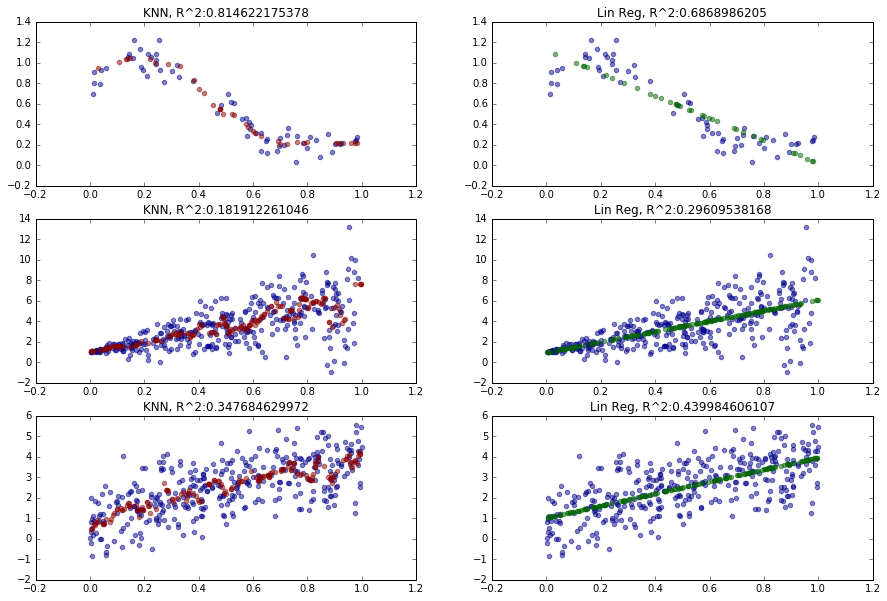The scatter plots shows the advantage of KNN, i.e., it tends to perform better when the data is not linear and when the shape of the distribution is odd. On the other hand, when the data is "linear", linear regression performs better than KNN (even with a high choice of $k$).

So when does the KNN model fail? The KNN overfits some of the data. This can be seen in model 2 and model 3 where a OLS estimations performas better that the KNN. When the nearest neighbours show extreme variation KNN also does poorly.

## Problem 3: Is the Best (Linear Model) Good Enough?¶

In this problem, we will specifically look at conditions under which linear regression excels or fails.

The datasets required for this problem is in the dataset directory. Each file in the dataset directory contains a one-dimensional data set, with the first column containing the independent variable X, and the second column containing the dependent variable Y.

### Part (a): Introduction to Residual Plots¶

• Read dataset_1_full.txt. Visualize the dataset and make some initial observations.
• For this data set, what can you say about the following linear fits:

1. slope = 0.4, intercept = 0.2
2. slope = 0.4, intercept = 4
3. linear regression model
• In each case, visualize the fit, compute the residuals, and make a residual plot of predicted values along with residuals, as well as a residual histogram. What do these plots reveal?
• Calculate the R^2 coefficient for all three fits. What do the erors reveal? How do they compare to the residual plots?

Solution:

In :
## Linear Model
def FitLinearModel(X, Y):
# Estimate the coefficients
beta1 = np.sum((X-np.mean(X)) * (Y-np.mean(Y)))/np.sum( (X-np.mean(X))**2)
beta0 = np.mean(Y) - beta1*np.mean(X)
return beta0, beta1

def PredictLinearModel(X, beta0, beta1):
Y = beta0 + beta1 * X
return Y

return np.sum((Y-PredictLinearModel(X, beta0, beta1))**2)

# Calculate TSS
def TSS(Y):
return np.sum((Y - np.mean(Y))**2)

# Calculate R^2
def R_Squared(Y, beta0, beta1):
return (1 - (RSS(Y, beta0, beta1) / TSS(Y)))

## Residual function
def calcResiduals(beta0, beta1):
Y_hat = PredictLinearModel(X, beta0, beta1)
return Y_hat - Y

# Ploting function
def plotIt(beta0, beta1):
plt.figure(figsize=(15, 10))
plt.plot(X, Y, 'ro', label='Data', markersize=10, color = "darkblue", alpha = 0.5)
plt.xlabel('X-Axis'); plt.ylabel('Y-Axis')
plt.title('Plot')
plt.grid()
plt.plot([X, X], [Y, beta0 + beta1 * X] , 'k', color = "darkgrey")
plt.legend()

In :
missing_df = pd.read_csv('./dataset/dataset_1_full.txt')

# get x and y vars
X = missing_df['x'].values
Y = missing_df['y'].values

In :
# 1. slope = 0.4, intercept = 0.2
plotIt(beta0=0.2, beta1=0.4)
# 2. slope = 0.4, intercept = 4
plotIt(beta0=4, beta1=0.4)
# 3. linear regression model
beta0_hat, beta1_hat = FitLinearModel(X, Y) # Estimate the coefficients
plotIt(beta0=beta0_hat, beta1=beta1_hat)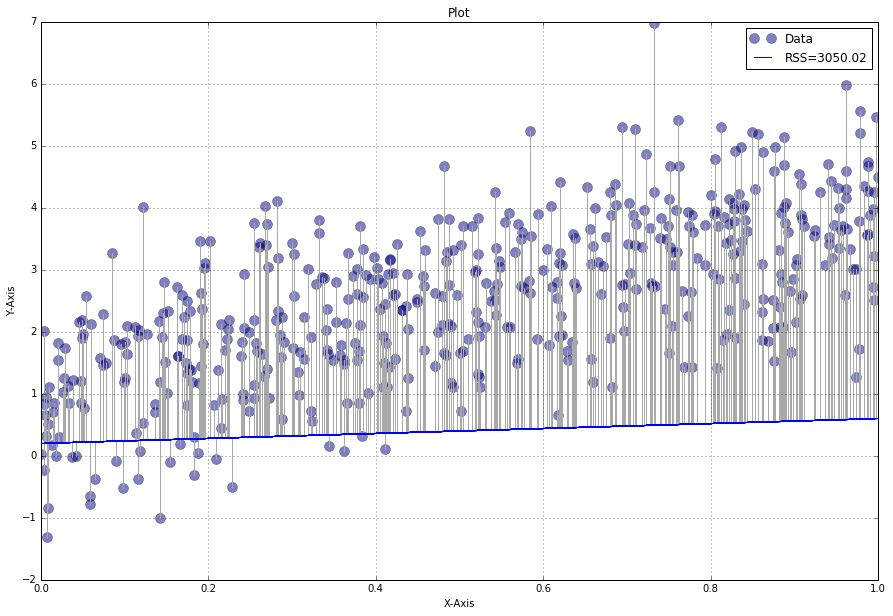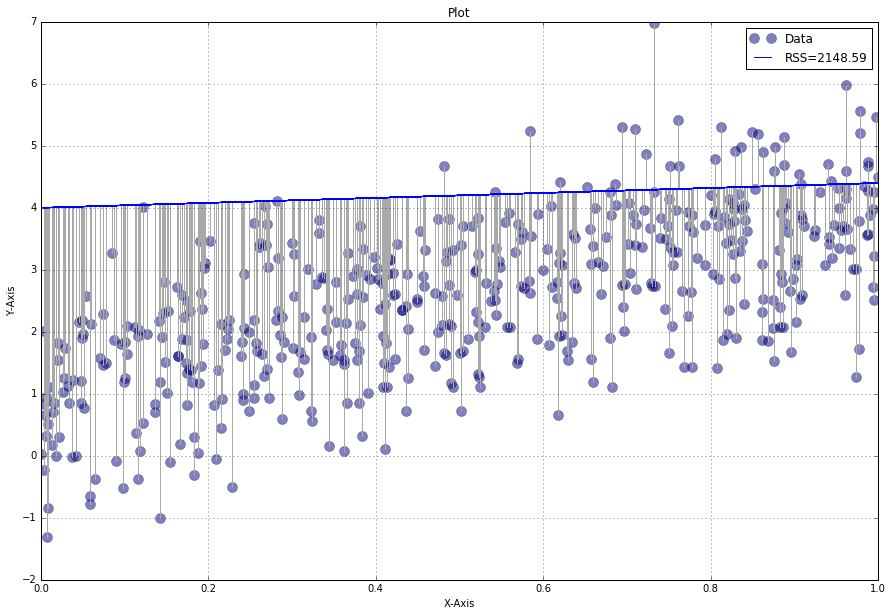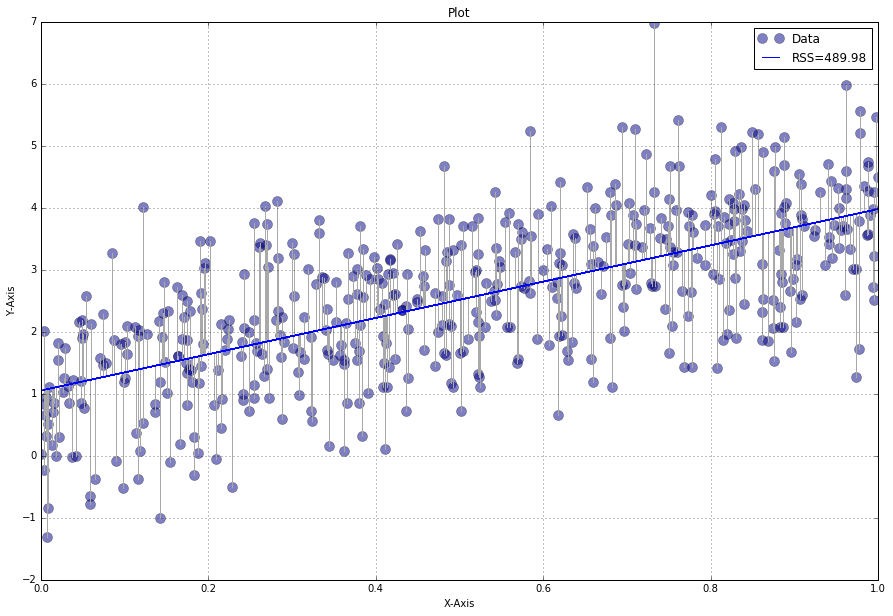In :
## Calculate residuals
residuals = calcResiduals(beta0=0.2, beta1=0.4)
plt.hist(residuals, bins=100, alpha=0.4, color="grey")
plt.axvline(x=0, linewidth=4)

residuals = calcResiduals(beta0=4, beta1=0.4)
plt.hist(residuals, bins=100, alpha=0.4, color="darkblue")
plt.axvline(x=0, linewidth=4)

residuals = calcResiduals(beta0=beta0_hat, beta1=beta1_hat)
plt.hist(residuals, bins=100, alpha=0.4, color="darkred")
plt.axvline(x=0, linewidth=4, color = "black")

Out:
<matplotlib.lines.Line2D at 0x13385e80>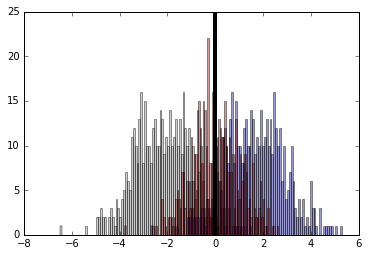In :
print R_Squared(Y, 0.2, 0.4)
print R_Squared(Y, 4, 0.4)
print R_Squared(Y, beta0_hat, beta1_hat)

-2.54429006179
-1.49677532281
0.430621521722


** Comment: **
Model 1 and model 2 both are very bad estimators of the underlying pattern in the data. Both the scatterplot as well as the histogram show, that they either systematicly under or overestimate the value of the underlying relationsship.

The OLS, i.e., model 3 is a much better estimator for the underlying relationship. It is able to explain 43% of the variation in the data. However, the bigger the x the larger the error in the estimation, i.e., there is a heteroscedastic error pattern present. That means that one of the OLS model assumtions is violated.

The reason that model 1 and model 2 deliever a negativ R^2 is, that the ^2 compares the fit of the chosen model with that of a horizontal straight line (the null hypothesis). If the chosen model fits worse than a horizontal line, then R2 is negative, i.e., R^2 is not always the square of anything, so it can have a negative value without violating any rules. R^2 is negative because model 1 and model 2 do not follow the trend of the data, so fits worse than a horizontal line.

### Part (b): What do Residual Plots Reveal?¶

• Read datasets dataset_2_full.txt through dataset_6_full.txt. In each case, visualize the fit, compute the residuals, and make a residual plot of predicted values along with residuals, as well as a residual histogram. What do these plots reveal about the fit of the model?
• Calculate the R^2 coefficient each fit. What do the erors reveal? How do they compare to the residual plots?
• Based on your analysis, form conjectures regarding the precise relationship between the residual plots and the fit of the linear regression model. Conjecture on the precise conditions under which linear regression model is an appropriate model for a given dataset.

Solution:

Dataset 2

In :
# Load dataset

# get x and y vars
X = df['x'].values
Y = df['y'].values

# linear regression model
beta0_hat, beta1_hat = FitLinearModel(X, Y) # Estimate the coefficients
plotIt(beta0=beta0_hat, beta1=beta1_hat)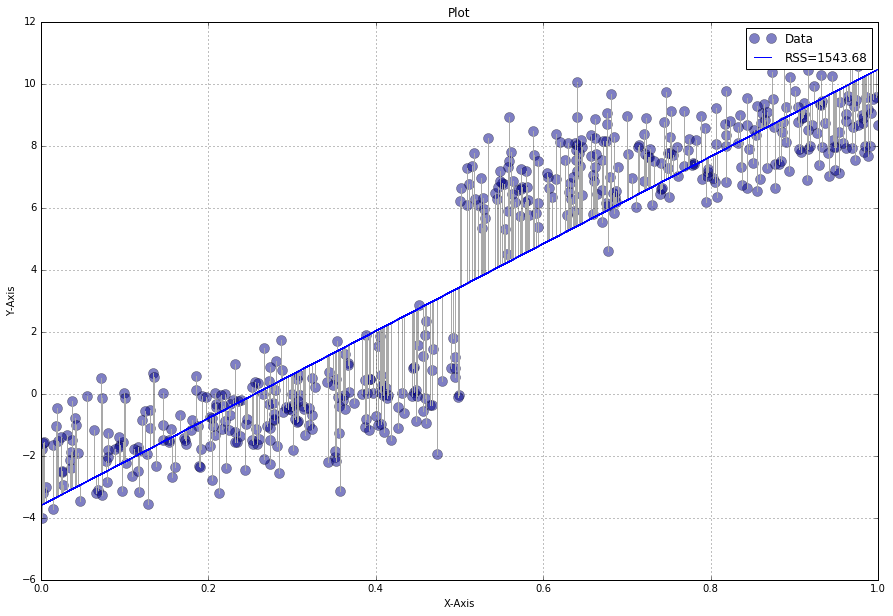In :
## Calculate residuals
residuals = calcResiduals(beta0=beta0_hat, beta1=beta1_hat)
plt.hist(residuals, bins=100, alpha=0.4, color="darkred")
plt.axvline(x=0, linewidth=4, color = "black")

print R_Squared(Y, beta0_hat, beta1_hat)

0.842957182013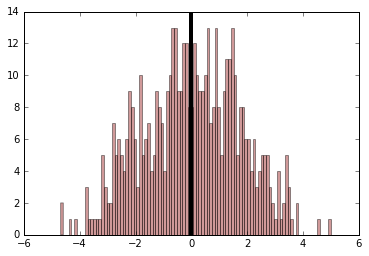** Comment: **
This model is able to explain 84% of the variation in the data. the histogram also looks very good. However, looking at the scatterplot reveals, that there is a shift or break at the 0.5 mark. It would be much better to create two seperate models one for < 0.5 and one for > 0.5.

Dataset 3

In :
# Load dataset

# get x and y vars
X = df['x'].values
Y = df['y'].values

# linear regression model
beta0_hat, beta1_hat = FitLinearModel(X, Y) # Estimate the coefficients
plotIt(beta0=beta0_hat, beta1=beta1_hat)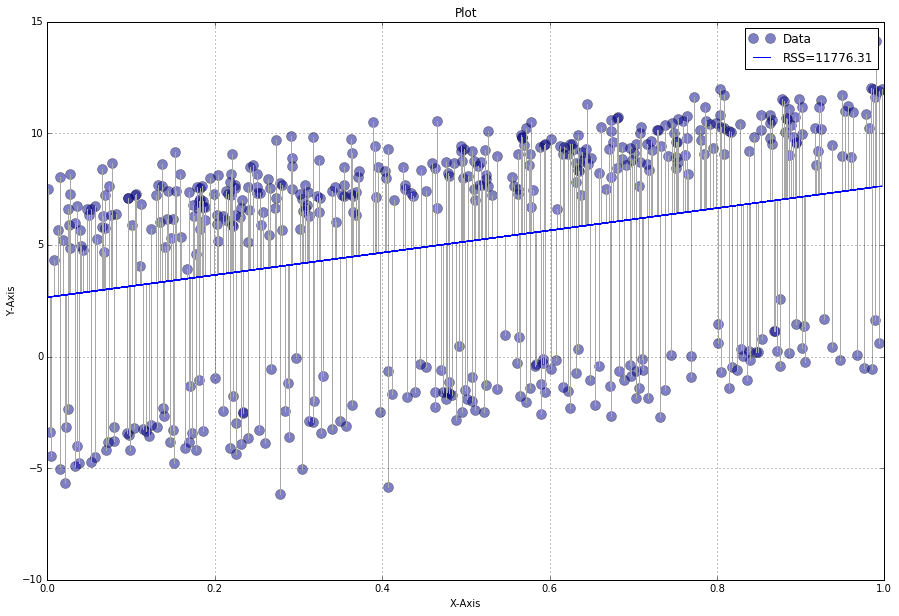In :
## Calculate residuals
residuals = calcResiduals(beta0=beta0_hat, beta1=beta1_hat)
plt.hist(residuals, bins=100, alpha=0.4, color="darkred")
plt.axvline(x=0, linewidth=4, color = "black")

print R_Squared(Y, beta0_hat, beta1_hat)

0.0779354128276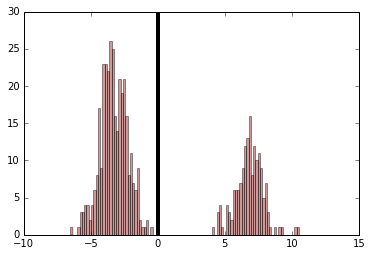** Comment: **
The histogram shows, that there are two distince error distribution, i.e., there is something else going on in the data. That means, that the errors are not normaly distributed. Results and interpretations of the model are either always under or always overestimated. This can also be seen by the fact that the model is only able to explain 7.8% of the variation in the data.

Dataset 4

In :
# Load dataset